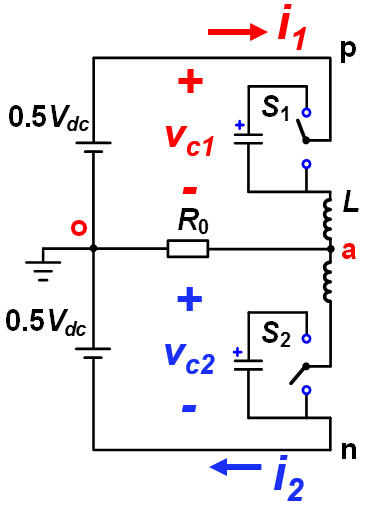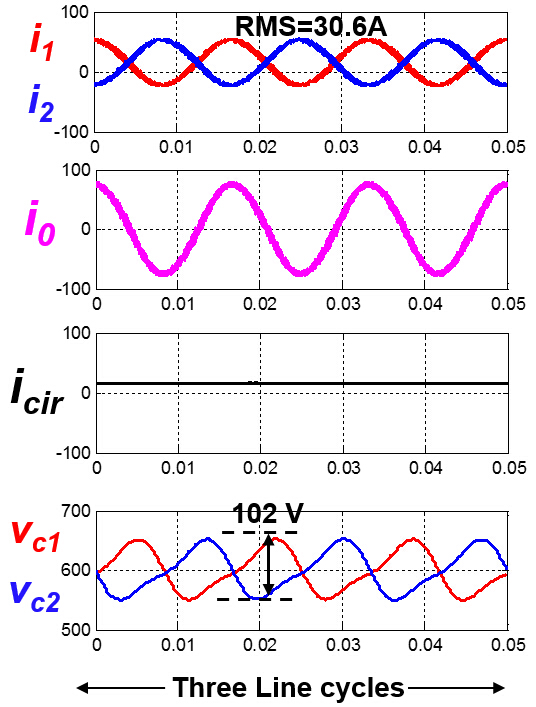LIBRARY

# Direct circulating current control and reducing cap voltage ripple of MMC

Year: 2015Fig. 1 : MMC topology
The modular multilevel converter (MMC) has attracted a lot of attention in the past decade. Because of its modularity and scalability features, the output of MMC can, in theory, be extended to arbitrary voltage levels. Therefore, it is an excellent choice in high power applications such as HVDC transmission, STATCOM, medium voltage motor drives, and so on. However, MMC has very bulky capacitors in each module, greatly increasing converter size and cost. Thus, figuring out how to reduce capacitance is one of the challenges for MMC. This paper focuses on reducing the cap voltage ripple by injecting different circulating currents.

First, the paper analyzes the basic working principle of MMC. Conventionally, the control law of MMC is given based on two assumptions: the cap voltage is constant; the voltage over the arm inductor is relatively small. As a result of these two assumptions, we can say that the output voltage doesn't exactly follow the reference, and that a 2nd order circulating current exists. In order to precisely control the output voltage and the circulating current, this paper provides a new control law. It has been shown that for eliminating the circulating current, this control law is approximately equivalent to the conventional closed loop control with a circulating current suppressing controller. Based on this new control law, the MMC' s operation is analyzed further, and the modulation index limitation is given when the circulating current is eliminated.

This paper next deals with the issue of reducing capacitor voltage ripple. When injecting different kinds of circulating current, the capacitor voltage ripple of the MMC should be different. According to the new control law, circulating current can be accurately controlled and thus a comparison of cases where different circulating currents are injected can be easily given. This paper attempts to provide an optimized injected circulating current that would bring the smallest capacitor voltage ripple.

The previous conclusions were reached based on a single phase MMC, but are also suitable for a three phase MMC. Additionally, the three phase MMC offers a common mode voltage, which can also be used to reduce cap voltage ripple. Therefore, there are more choices and possibilities for a three phase MMC; for example, a high frequency common mode voltage and circulating current could be injected at the same time. In the conclusion, this paper provides an analysis of the aforementioned method based on the new control law.Fig. 2. Circulating current and cap voltage ripple of MMC